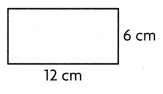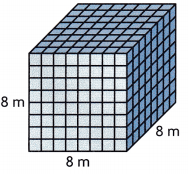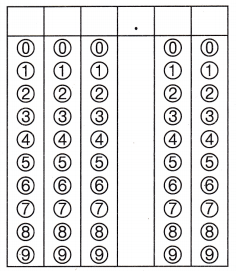Refer to our Texas Go Math Grade 5 Answer Key Pdf to score good marks in the exams. Test yourself by practicing the problems from Texas Go Math Grade 5 Module 9 Assessment Answer Key.

Vocabulary

Choose the best term from the box.

Vocabulary
cubic units
formula
volume

Question 1.
_Volume____ is the amount of space occupied by a solid figure. (p. 355)
Volume,

Explanation:
As we know in math, volume is defined as the 3 dimensional space
enclosed by a boundary or occupied by an object.

Question 2.
A ___formula______ is an equation that expresses a mathematical rule. (p. 349)
formula,

Explanation:
A formula can also be called an equation that expresses
a relationship between two or more variables.

Concepts and Skill

Use formulas to find the perimeter and area. (TEKS 5.4.H)

Question 3.Perimeter: _______________
Area: ______________

Question 4.Perimeter: ____20 ft___________
Area: _____25 square ft_________
Perimeter: 20 ft,
Area: 25 square ft,

Explanation:
Given square with side 5 feet as we know perimeter of square is
4 X side, so perimeter = 4 X 5 feet = 20 ft and area of square is
side X side = 5 fet X 5 ft = 25 square ft.

Use formulas to find the volume. (TEKS 5.4.G, 5.4.H)

Question 5.Volume: _____512 cubic m________
Volume is 512 cubic m,

Explanation:
Given rectangular cube with edge 8 m,
so volume is edge X edge X edge =
8 m X 8 m X 8 m = 512 cubic m.

Question 6.Volume: _____________
Volume is 1287 cubic cm,

Explanation:
Given rectangular cuboid with length 11 cm, width 9 cm and
height 13 cm, so volume is length X width X height =
11 cm X 9 cm X 13 cm = 1287 cubic cm.

Question 7.
Find the area of the polygon. Show how you find your answer. (TEKS 5.4.H)Area: _____52 square cm________
Area of the polygon is 52 square cm,Explanation:
Given to find area of the polygon but is irregular polygon we divide into
regular shapes of two rectangles as shown above one with 8 cm length and
width 5 cm other with length 6 cm and width 2 cm then we add to get
the area of total polygon, so first rectangle area is 8 cm X 5 cm = 40 square cm,
other rectangle area is 6 cm X 2 cm = 12 square cm,
total area is 40 square cm + 12 square cm = 52 square cm.

Question 8.
Which equation shows how to find the volume of the model? (TEKS 5.4.G, 5.4.H)(A) 8 × 5 = 40
(B) (2 × 8) + (2 × 5) = 26
(C) 8 × 5 × 4 = 160
(D) 8 + 5 + 4 = 17
(C) 8 × 5 × 4 = 160

Explanation:
Given model shape is regular cuboid with length 8 cm,
width 5 cm and height 4 cm, so equation showing
to find the volume of the model as V = length X width X height is
V = 8 X 5 X 4 = 160 which matches with (C).

Question 9.
Sanaya has a rectangular bulletin board that is 3 feet long and 2 feet wide.
She wants to cover the board with felt and add a satin ribbon around
the border of the bulletin board.
How much felt and ribbon does Sanaya need? (TEKS 5.4.H)
(A) 6 feet of felt, 10 feet of ribbon
(B) 6 sq feet of felt, 10 feet of ribbon
(C) 10 sq feet of felt, 6 feet of ribbon
(D) 6 sq feet of felt, 5 feet of ribbon
(B) 6 sq feet of felt, 10 feet of ribbon,

Explanation:
Given Sanaya has a rectangular bulletin board that is 3 feet long and 2 feet wide.
She wants to cover the board with felt and add a satin ribbon around
the border of the bulletin board. So felt and ribbon does Sanaya need is
as felt is base area A = long X wide, A= 3 feet X 2 feet = 6 sq feet,
and ribbon is perimeter = 2 (long + wide) = 2 X (3feet + 2 feet) = 3 X 5 feet = 10 feet,
therefore Sanaya needs 6 sq feet of felt, 10 feet of ribbon which matches with (B).

Question 10.
Hicham buys a rectangular sheet of plywood that measures 18 square meters.
The sheet of plywood is 3 meters long.
What is the perimeter of the sheet of plywood? (TEKS 5.4.H)
(A) 9 meters
(B) 6 meters
(C) 18 meters
(D) 18 square meters
(C) 18 meters,

Explanation:
Given Hicham buys a rectangular sheet of plywood that measures 18 square meters.
The sheet of plywood is 3 meters long. To find the perimeter of the sheet of plywood
we need first width as Area = long X wide, 18 square meters = 3 meters X w,
w = 18 ÷ 3 meters = 6 meters, Now the perimeter of the sheet of plywood is
2( long + wide) = 2 X (3 meters + 6 meters) = 2 X (9 meters) = 18 meters
which matches with (C).

Question 11.
Melinda is making a tin box shaped like a rectangular prism.
For the base, Melinda cuts out a square with a side length of 12 inches.
If Melinda wants the volume of the tin box to be 1,440 cubic inches,
how tall should the box be? (TEKS 5.4.H )
(A) 12 inches
(B) 10 inches
(C) 6 inches
(D) 8 inches
(B) 10 inches,

Explanation:
Given Melinda is making a tin box shaped like a rectangular prism.
For the base, Melinda cuts out a square with a side length of 12 inches.
If Melinda wants the volume of the tin box to be 1,440 cubic inches,
the height of the box must be given length is 12 inches and
width also 12 inches(square) as we know volume = length X width X height ,
1,440 cubic inches = 12 inches X 12 inches X height/tall,
1,440 cubic inches = 144 square inches X height/tall,
therefore height/tall = 1,440 cubic inches ÷ 144 square inches,
height/tall = 10 inches which matches with (B).

Question 12.
Deval has a rectangular patio that is 15 feet long and 5 feet wide.
If Deval uses square tiles with a side length of 1 foot,
how many tiles will he need to cover the patio? (TEKS 5.4.H)